Group Delay

The signal input to the filter causes a delay when output. How much delay time each frequency of the output signal has with respect to the input is referred to as the group delay characteristic. In concrete, the group delay is obtained by integrating the phase characteristic in terms of the frequency and used to evaluate the characteristic of a filter circuit. With this characteristic, the value (delay time) depends on the filter circuit and frequency. When using a filter with different delay time for the frequency, waveform distortion arises in the output signal with respect to the input signal. Therefore, FFT analyzers correct this value, remove waveform distortion, and display an exact waveform.

Harmonic Distortion

Vibration waveform observed in the mechanical vibration system usually contains various harmonic components in addition to the fundamental component. When a sine wave is input to a transmission system, harmonic components, referred to as distortion components, of the input signal appear in the output signal because of the non-linear characteristic of the transmission system. By focusing this distortion, harmonic components of vibration waveform or output signal are analyzed and the vibration characteristics and the fidelity of the transmission system are considered.

When the waveform under observation, generally the output waveform, consists of fundamental frequency f1, 2nd order harmonic frequency f2, 3rd order harmonic frequency f3, and other harmonic components and if the RMS value of each component is |E1|, |E2|, |E3|, ..., the total harmonic distortion is defined as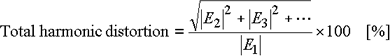Using the power spectrum of each frequency component (p1, p2, p3, ...), the total harmonic distortion becomes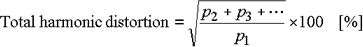When the harmonic distortion in the n-th harmonic component is focused, the following n-th order relative harmonic content is used: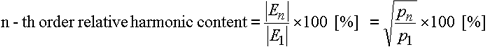Hilbert Transform

The Hilbert transform, g (t), and the inverse Hilbert transform of real function f (t) are defined by expressions (1) and (2), respectively.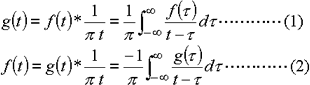The * mark denotes convolution.

Using Hilbert transform g (t) of real function f (t), analysis signal (complex number) Z (t) is defined by the following expression (3).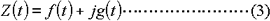Since Z (t) is a complex number, it can be represented as a vector.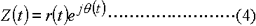where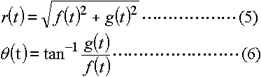r (t) is referred to as the amplitude (envelope) of f (t) and θ (T) as the instantaneous phase. Therefore, any real function f (t) can be represented by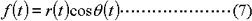Thus, the use of the Hilbert transform makes it possible to obtain the envelope of f(t) and calculate the logarithmic damping ratio (and then the damping factor).

The envelope represents the temporal variation of the instantaneous energy of a system. In addition, f(t) can be observed not only by the amplitude but also by another parameter, i.e., the instantaneous phase.

When θ (t) is differentiated,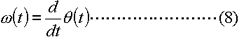it can be recognized as the instantaneous angular frequency.

The X axis is assigned the frequency and the Y axis is assigned a logarithmic scale where 1 V2rms corresponds to 0 dB Vrms.

Impulse Response

Response h (t) output by the system when unit impulse δ (t) is applied to it is referred to as impulse response. Impulse response is a system characteristic expressed in the time domain. On the other hand, a system characteristic expressed in the frequency domain is referred to as the transfer function.

If the impulse response of a system is known, output y (t) when x (t) is input to the system can be calculated through convolution of input x (t) and impulse response.

FFT analyzers from Ono Sokki perform the inverse Fourier transform of the frequency response function to obtain the impulse response.

Inverse Fourier Transform

The relationship between the Fourier transform and the inverse Fourier transform is shown below.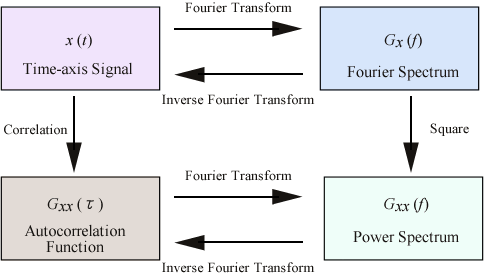The inverse Fourier transform of the cross spectrum is the cross-correlation function. The inverse Fourier transform of the frequency response function is the impulse response.

Isolation

Insulation of electrical signals is referred to as isolation. It is required for measurement when the common line of the signal source differs from the ground potential. Measurement is possible when the difference between the signal common line and the ground potential, common mode voltage, is small. When it is 100 V or higher, measurement is not possible because of excessive input. In this case, an isolation amplifier or the like may be used and, as for the FFT analyzer, the analog input section including the amplifier, anti-aliasing filter, and A-D converter is isolated from the chassis ground using a photo-coupler on a channel basis. Since the digital circuit and analog circuit are insulated, isolation is advantageous when eliminating the ground loop or removing connection with the common line of the signal source.

Liftered Envelope

The envelope of the power spectrum is obtained by performing inverse Fourier transform of the short quefrency. The liftered envelope is specific to the signal transmission system and does not depend on the spectrum of the input signal.

Applications of the liftered envelope include extraction of the fundamental frequency and power spectrum envelope from sound waves, bio-waves, etc.